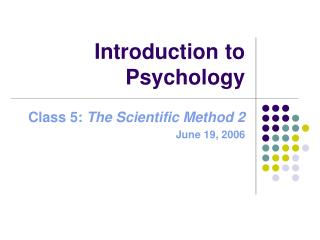# Introduction to Psychology - PowerPoint PPT PresentationDownload PresentationIntroduction to Psychology

Introduction to Psychology
Download Presentation## Introduction to Psychology

- - - - - - - - - - - - - - - - - - - - - - - - - - - E N D - - - - - - - - - - - - - - - - - - - - - - - - - - -
##### Presentation Transcript

1. Introduction to Psychology Class 5: The Scientific Method 2 June 19, 2006

2. Measures of Central Tendency Consider this sample: 3 3 5 6 7 2 8 9 5 5 3 30 Mean = 86/12 = 7.17 Mode = 3 and 5 (bimodal) Median = (5 + 5)/2 = 5 Outlier

3. Correlation • Answers the question: what is the linear relationship between two variables • Consider amount of coffee and performance on an exam in a sample of coffee drinkers as an example Number of cups Exam score 1.0 90 1.5 89 2.0 95 2.5 95 3.0 99

4. Graph The Correlation between Performance and Amount of Coffee r = +.92

5. NUMBER VALUE: 0 to 1 and SIGN: + or – r = + .63 r = + .89 r = + 1.0 r = + .28 r = ~ 0 r = - .91

6. Limitations r = - . 18 Works for LINEAR relationships only.

7. SCORE COFFEE EXPECTATION Correlation is NOT causation.

8. Experimentation Control group Random Assignment Independent variable/s Dependent variable/s Experimental group Statistical tests

9. Example • Design an experiment to test the effect of drinking coffee prior to an exam on performance and expectations in agroup of coffee drinkers • ONE independent variable  Caffeinated or decaf drink (2 levels) • TWO dependent variables:  Actual exam score  Expected exam score

10. Procedure • Give subjects instructions to not drink any coffee before the experimental session • Randomly assign coffee drinkers to control (n=20) and experimental group (n=20) • Make participants in the control group drink a cup of decaf and those in the experimental group drink a cup of coffee, but tell both that it is coffee • Give them all the same exam under the same conditions • After they finish, ask them to estimate their score on the exam • Then actually score their exams • Compute the means for each variable and group

11. Results Actual score Expected score Coffee 85.1 Coffee 90.8 Decaf 75.9 Decaf 91.2 Insignificant difference * Significant difference * * Determined statistically

12. Design an experiment! • Group activity • Come up with an experiment that has:  1 independent variable  1 dependent variable • Define your sample • Explain your procedure • State your hypothesis • Present your experiment